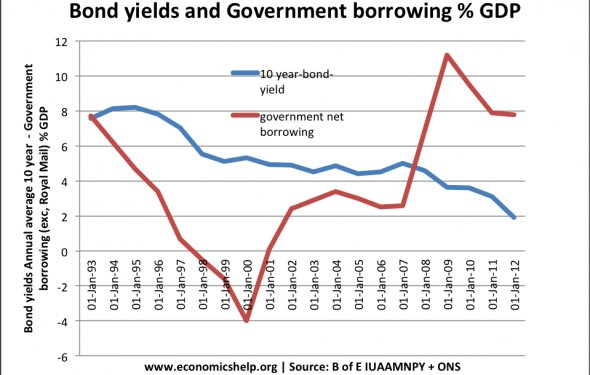# Bond yields and interest rates

Bond Basics: Bond Prices and Yield to Maturity
June 22, 2017 – 03:20 pmThe market prices bonds based on the bond's particular characteristics. A bond's price changes on a daily basis, just like that of any other publicly-traded security, where supply and demand in any given moment determines that observed price. But there is a logic to how bonds are valued. Up to this point, we've talked about bonds as if every investor holds them to maturity. It's true that if you do this you're guaranteed to get your principal back plus interest; however, a bond does not have to be held to maturity. At any time, a bondholder can sell their bonds in the open market, where the price can fluctuate, sometimes dramatically.

The price of a bond changes in response to changes in interest rates in the economy. This is due to the fact that for a fixed-rate bond, the issuer has promised to pay a coupon based on the face value of the bond – so for a \$1, 000 par, 10% annual coupon bond, the issuer will pay the bondholder \$100 each year.

Say that prevailing interest rates are also 10% at the time that this bond is issued, as determined by the rate on a short-term government bond. An investor would be indifferent investing in the corporate bond or the government bond since both would return \$100. Imagine a little while later, however, that the economy has taken a turn for the worse and interest rates drop to 5%. Now, the investor can only receive \$50 from the government bond, but would still receive \$100 from the corporate bond. This makes the corporate bond much more attractive, and so investors in the market will bid up the price of the bond until it trades at a premium that equalizes the prevailing interest rate environment – in this case the bond will trade at a price of \$2, 000 so that the \$100 coupon represents 5%. Likewise, if interest rates soared to 15%, then an investor could make \$150 from the government bond and would not pay \$1, 000 to earn just \$100. This bond would be sold until it reached a price that equalized the yields, in this case to a price of \$666.67.

This is why the famous statement that a bond’s price varies inversely with interest rates works. When interest rates go up, bond prices fall in order to have the effect of equalizing the interest rate on the bond with prevailing rates, and vice versa. Another way of illustrating this concept is to consider what the yield on our bond would be given a price change, instead of given an interest rate change. For example, if the price were to go down from \$1, 000 to \$800, then the yield goes up to 12.5%. This happens because you are getting the same guaranteed \$100 on an asset that is worth \$800 (\$100/\$800). Conversely, if the bond goes up in price to \$1, 200, the yield shrinks to 8.33% (\$100/\$1, 200).

The yield-to-maturity (YTM) of a bond is another way of considering a bond’s price. YTM is the total return anticipated on a bond if the bond is held until the end of its lifetime. Yield to maturity is considered a long-term bond yield, but is expressed as an annual rate. In other words, it is the internal rate of return of an investment in a bond if the investor holds the bond until maturity and if all payments are made as scheduled. YTM is a complex calculation but is quite useful as a concept evaluating the attractiveness of one bond relative to other bonds of different coupon and maturity in the market. The formula for YTM involves solving for the interest rate in the following equation, which is no easy task, and therefore most bond investors interested in YTM will use a computer:

We can also measure the anticipated changes in bond prices given a change in interest rates with a measure knows as the duration of a bond. Duration is expressed in units of number of years since it originally referred to zero-coupon bonds, whose duration is its maturity. For practical purposes, however, duration represents the price change in a bond given a 1% change in interest rates. We call this second, more practical definition the modified duration of a bond. Duration can be calculated to determine the price sensitivity to interest rate changes of a single bond, or for a portfolio of many bonds. In general, bonds with long maturities, and also bonds with low coupons have the greatest sensitivity to interest rate changes. A bond’s duration is not a linear risk measure, meaning that as prices and rates change, the duration itself changes, and convexity measures this relationship.

Source: www.investopedia.com
Related Posts